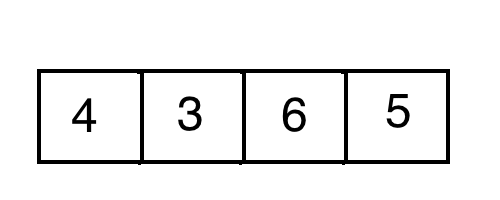# Insertion Sort

Insertion Sort is another sorting algorithm that we can use to sort arrays. Going back to the statistics package example discussed in the previous chapter, we can use Insertion Sort to sort our array of integers so that we can find the median value.

##### How it works

As with Selection Sort, Insertion Sort uses loops to iterate over the array. However, there is an important difference: while Selection Sort searches for the smallest element on each iteration, Insertion Sort immediately puts each element into its designated position as it iterates over the array.##### What it looks like

Here is an example of what an Insertion Sort algorithm looks like:

``````// Note: In some cases the list may be sorted in reverse order.

public class InsertionSort extends ConsoleProgram
{
public void run()
{
// Create our array of integers to sort
int[] intsToSort = {1, 5, 6, 3};

// We start at `1` instead of `0`
// because first value is already sorted
for(int i = 1; i < intsToSort.length; i++)
{
int currNum = intsToSort[i];

// Shift element into designated position
int currIndex = i-1;
while(currIndex > -1 && intsToSort[currIndex] > currNum)
{
intsToSort[currIndex+1] = intsToSort[currIndex];
currIndex--;
}
intsToSort[currIndex+1] = currNum;
}
}
}
``````